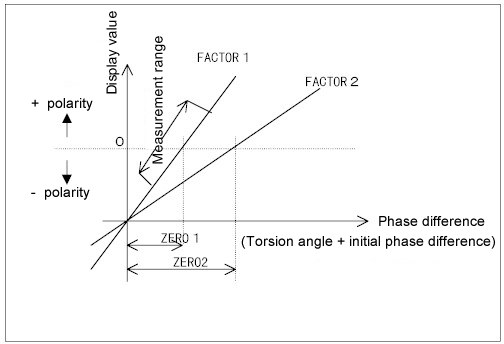Torquemeter FAQRelationship between Factor and Zero (temperature characteristic)A torque detector detects a torsion angle of a torsion bar as the phase difference and the value that is converted to a torque value is displayed on the indicator.

In the following diagram, the phase difference is assigned to the X axis and the torque display value is assigned to the Y axis.

(1) Factor

A factor indicates a ratio between the phase difference and the display value and is equivalent to the inclination of the straight line. If the factor is increased, the inclination increases. In the diagram below, FACTOR1>FACTOR2A.

The torque detector determines the factor by performing calibration and test individually. The determined factor value is indicated on the nameplate of the torque detector.

(2) Zero

Without load, the initial phase difference is set to about 180 degrees. Set the "Zero" value so that 0 is displayed at this point. If the torque is applied on the axis and the torsion bar is slightly twisted, the phase difference is increased corresponding to the torsion angle θ.

(180 deg+θ =+torque)

When a reverse torque (minus torque) is applied, the phase difference decreases (180 deg -θ).  ± Polarity is applied to the display and voltage output according to the direction of the torque.

(3) Relationship between FACTOR and ZERO

As shown below, since the "phase difference zero" is not equal to "display zero", a change of the FACTOR results in the change of the ZERO setting value. Since the factor varies according to the torque detector, it is necessary to set "Factor" and "Zero" at the initial use.(4) Influence of temperature

For measurement of higher precision, an influence of temperature can be corrected. If the temperature changes dramatically, the spring constant of the torsion bar changes, resulting in a slight change of the phase difference for the torque value.

The higher the temperature, the larger direction that the torque is displayed. A temperature coefficient is indicated in the torque detector instruction manual as shown below.

(E.g.)Temperature characteristic 0.03%/℃

A temperature influence can be corrected by the formula (5). Since there is a change of Zero due to the factor of the entire torque detector, make Zero adjustment after the temperature of the torque detector is stabilized by performing warm-up before starting the measurement.

(5) How to compensate temperature characteristic

The nameplate of a detector indicates the FACTOR, RANGE (or CAPACITY), and TEMP (temperature of the torsion bar) T1 at the in-house test. Since temperature T2 of the torsion bar at measurement is almost the same as the temperature at the end of the axis, perform measurement when the temperature is stable.

Use the following formula to correct the measured value after completion of measurement.

(E.g.)
T1=15ºC, T2=45ºC, temperature coefficient 0.003%/ºC measured value 25.00N•m
Actual value= (Measured value) / {1+0.03 (T2-T1) / 100}=24.78 N•m

For the details, refer to the torque detector instruction manual.

Revised:2007/09/18